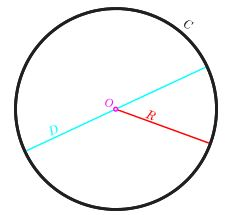Courses

# Theory - Area of the Circle is half the product of its Circumference & Radius, Class 9 Math Class 9 Notes | EduRev

## Mathematics (Maths) Class 9

Created by: Indu Gupta

## Class 9 : Theory - Area of the Circle is half the product of its Circumference & Radius, Class 9 Math Class 9 Notes | EduRev

The document Theory - Area of the Circle is half the product of its Circumference & Radius, Class 9 Math Class 9 Notes | EduRev is a part of the Class 9 Course Mathematics (Maths) Class 9.
All you need of Class 9 at this link: Class 9

Objective:

To give a suggestive demonstration of the formula that the area of the circle is half the product of its circumference and radius.

Definition:

Circle:  It is the set of all points in a plane that are at a given distance from a given point, the centre.

A circle with circumference (C) in black, diameter (D) in cyan, radius (R) in red, and centre (O) in magenta is below:Related Terms:

Center: A point inside the circle. All points on the circle are equidistant (same distance) from the center point.
Radius: The radius is the distance from the center to any point on the circle. It is half the diameter.
Chord: A segment of a straight line joining two points on a circle.
Diameter: The length of any chord passing through the center. It is twice the radius.
Circumference: The circumference is the perimeter of the circle. It is 2πr.
Area: Area of the region enclosed by the circle. It is πr2.

Properties of a circle:

• The radius perpendicular to a chord bisects the chord.

• The diameter of a circle is the longest chord.

## Mathematics (Maths) Class 9

192 videos|230 docs|82 tests

,

,

,

,

,

,

,

,

,

,

,

,

,

,

,

,

,

,

,

,

,

,

,

,

;